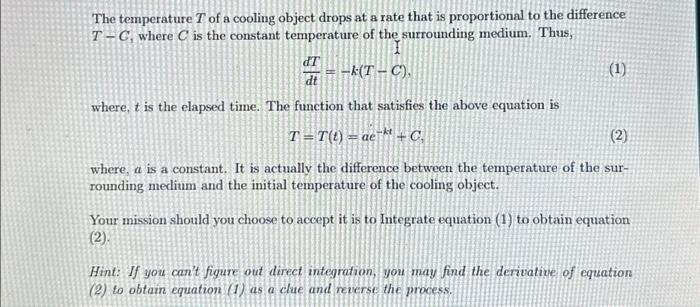Home / Expert Answers / Calculus / the-temperature-t-of-a-cooling-object-drops-at-a-rate-that-is-proportional-to-the-difference-t-c-wh-pa763

# (Solved): The temperature T of a cooling object drops at a rate that is proportional to the difference T-C, wh ...

The temperature T of a cooling object drops at a rate that is proportional to the difference T-C, where C is the constant temperature of the surrounding medium. Thus,
dT/dt=-k(t-c), (1)

where, t is the elapsed time. The function that satisfies the above equation is
T = T(t) = ae^-kt +C, (2)

where, a is a constant. It is actually the difference between the temperature of the surrounding medium and the initial temperature of the cooling object. Your mission should you choose to accept it is to Integrate equation (1) to obtain equation (2).The temperature of a cooling object drops at a rate that is proportional to the difference , where is the constant temperature of the surrounding medium. Thus, where, is the elapsed time. The function that satisfies the above equation is where, is a constant. It is actually the difference between the temperature of the surrounding medium and the initial temperature of the cooling object. Your mission should you choose to accept it is to Integrate equation (1) to obtain equation Hint: If you can't figure out direct integration, you may find the derivative of equation (2) to obtain equation (1) as a clue and reverse the process.

We have an Answer from Expert